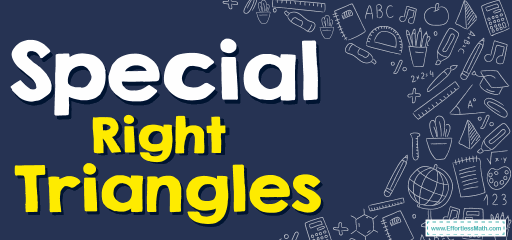# Special Right TrianglesThe special right triangle is a right triangle whose sides are in a particular ratio. According to the special right triangle rules, it is not necessary to use the Pythagorean theorem to get the size of a side.

## A step-by-step guide to solving Special Right Triangles

• Two special right triangles are $$45^{\circ}-45^{\circ}-90^{\circ}$$ and $$30^{\circ}-60^{\circ}-90^{\circ}$$ triangles.
• In a special $$45^{\circ}-45^{\circ}-90^{\circ}$$ triangle, the three angles are $$45^{\circ}, 45^{\circ}$$ and $$90^{\circ}$$. The lengths of the sides of this triangle are in the ratio of $$1:1:\sqrt{2}$$.
• In a special triangle $$30^{\circ}-60^{\circ}-90^{\circ}$$, the three angles are $$30^{\circ}-60^{\circ}-90^{\circ}$$. The lengths of this triangle are in the ratio of $$1:\sqrt{3}:2$$.

### Special Right Triangles – Example 1:

Find the length of the hypotenuse of a right triangle if the length of the other two sides are both 4 inches.

Best Algebra Prep Resource

Solution:

This is a right triangle with two equal sides. Therefore, it must be a $$45^{\circ}-45^{\circ}-90^{\circ}$$ triangle. Two equivalent sides are 4 inches. The ratio of sides: $$x:x:x\sqrt{2}$$. The length of the hypotenuse is $$4\sqrt{2}$$ inches. $$x:x:x\sqrt{2}→4:4:4\sqrt{2}$$

### Special Right Triangles – Example 2:

The length of the hypotenuse of a triangle is 6 inches. What are the lengths of the other two sides if one angle of the triangle is $$30^{\circ}$$?

Solution:

The hypotenuse is 6 inches and the triangle is a $$30^°-60^°-90^°$$ triangle. Then, one side of the triangle is 3 (it’s half the side of the hypotenuse) and the other side is $$3\sqrt{3}$$. (it’s the smallest side times $$\sqrt{3}$$) $$x:x\sqrt{3}:2x→x=3→x:x\sqrt{3}:2x=3:3\sqrt{3}:6$$

## Exercises for Special Right Triangles

### Find the value of x and y in each triangle.

1-

2-

3-

4-

5-

6-

1. $$\color{blue}{x=1,y=\frac{\sqrt{3}}{2}}$$
2. $$\color{blue}{x=4\sqrt{3},y=8\sqrt{3}}$$
3. $$\color{blue}{x=8\sqrt{2}}$$
4. $$\color{blue}{x=3\sqrt{2},y=3\sqrt{2}}$$
5. $$\color{blue}{x=3\sqrt{3},y=6\sqrt{3}}$$
6. $$\color{blue}{x=4\,y=2\sqrt{2}}$$

The Absolute Best Book for the Algebra Test

### What people say about "Special Right Triangles - Effortless Math: We Help Students Learn to LOVE Mathematics"?

No one replied yet.

X
51% OFF

Limited time only!

Save Over 51%

SAVE $15 It was$29.99 now it is \$14.99